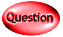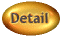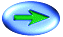3. Which of the following describes long run equilibrium for a firm in monopolistic competition with free entry?
1. Marginal Revenue = Average Total Cost, Price > Marginal Cost.
2. Price > Average Total Cost, Price = Marginal Cost.
3. Price > Average Total Cost, Marginal Revenue = Marginal Cost.
4. Price = Average Total Cost, Marginal Revenue > Marginal Cost.
5. Price = Average Total Cost, Price > Marginal Cost.